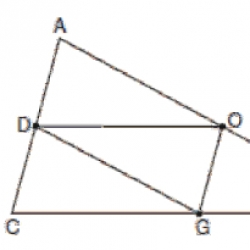Mid-point theorem
 1) In the diagram below of ACT, D is the midpoint of AC, O is the midpoint of AT , and G is the midpoint of CT.If AC = 10 ,AT=18, and CT=22, what is the perimeter of parallelogram CDOG ?21 32 24 54

Cite this Simulator: